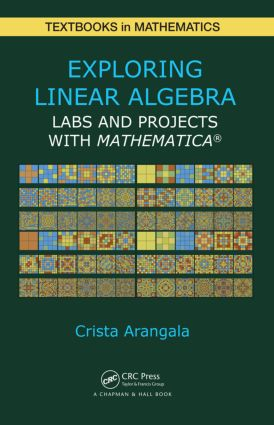# Exploring Linear Algebra

## Labs and Projects with Mathematica ®, 1st Edition

Chapman and Hall/CRC

151 pages | 38 B/W Illus.

##### Purchasing Options:\$ = USD
Hardback: 9781482241495
pub: 2014-11-13
\$59.95
x

FREE Standard Shipping!

### Description

Exploring Linear Algebra: Labs and Projects with Mathematica® is a hands-on lab manual for daily use in the classroom. Each lab includes exercises, theorems, and problems that guide your students on an exploration of linear algebra.

The exercises section integrates problems, technology, Mathematica® visualization, and Mathematica CDFs, enabling students to discover the theory and applications of linear algebra in a meaningful way. The theorems and problems section presents the theoretical aspects of linear algebra. Students are encouraged to discover the truth of each theorem and problem, to move toward proving (or disproving) each statement, and to present their results to their peers.

Each chapter also contains a project set consisting of application-driven projects that emphasize the material in the chapter. Students can use these projects as the basis for further undergraduate research.

### Reviews

"The presented book provides readers with a set of interesting exercises and problems regarding linear algebra. The book covers the scope of an undergraduate course … excellent supplementary material for a linear algebra course …"

Zentralblatt MATH 1320

Matrix Operations

Lab 0: An Introduction to Mathematica

Lab 1: Matrix Basics and Operations

Lab 2: A Matrix Representation of Linear Systems

Lab 3: Powers, Inverses, and Special Matrices

Lab 4: Graph Theory and Adjacency Matrices

Lab 5: Permutations and Determinants

Lab 6: 4 x 4 Determinants and Beyond

Project Set 1

Invertibility

Lab 7: Singular or Nonsingular? Why Singularity Matters

Lab 8: Mod It Out, Matrices with Entries in Zp

Lab 9: It’s a Complex World

Lab 10: Declaring Independence: Is It Linear?

Project Set 2

Vector Spaces

Lab 11: Vector Spaces and Subspaces

Lab 12: Basing It All on Just a Few Vectors

Lab 13: Linear Transformations

Lab 14: Eigenvalues and Eigenspaces

Lab 15: Markov Chains, An Application of Eigenvalues

Project Set 3

Orthogonality

Lab 16: Inner Product Spaces

Lab 17: The Geometry of Vector and Inner Product Spaces

Lab 18: Orthogonal Matrices, QR Decomposition, and Least Squares Regression

Lab 19: Symmetric Matrices and Quadratic Forms

Project Set 4

Matrix Decomposition with Applications

Lab 20: Singular Value Decomposition (SVD)

Lab 21: Cholesky Decomposition and Its Application to Statistics

Lab 22: Jordan Canonical Form

Project Set 5

Applications to Differential Equations

Lab 23: Linear Differential Equations

Lab 24: Higher-Order Linear Differential Equations

Lab 25: Phase Portraits, Using the Jacobian Matrix to Look Closer at Equilibria

Project Set 6

Mathematica Demonstrations and References

Index

### Subject Categories

##### BISAC Subject Codes/Headings:
MAT002000
MATHEMATICS / Algebra / General
MAT003000
MATHEMATICS / Applied